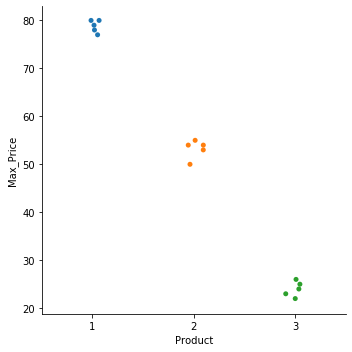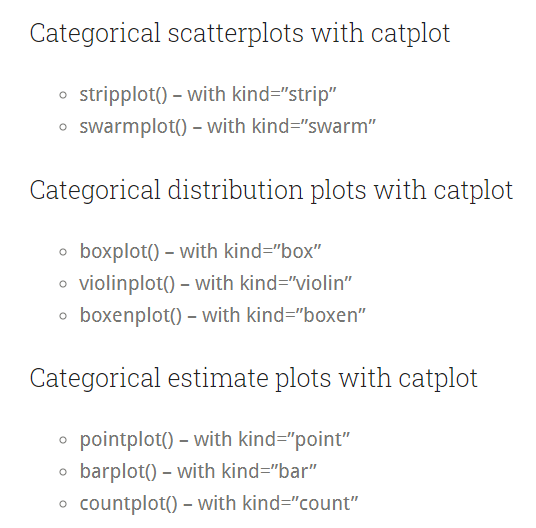# Catplot in Seaborn

There are many types of plots available in the seaborn module. However, when working with categorical values, we may need a constant method to plot the data since different plot functions work differently. This method is not consistent with the data. The `catplot()` function of this module is used to work with categorical data efficiently. This function was built to improve the `factorplot()` function in the recent versions of the seaborn module.

It allows us to work with categorical values efficiently, and we can plot the data into eight different types of graphs specified by the `kind` parameter. The `catplot()` function returns a `FacetGrid()` type object so it can be utilized efficiently to plot graphs for multiple features on the same figure.

It is simple to use the `catplot()` function. See the following code.

``````import pandas as pd
import matplotlib.pyplot as plt
import seaborn as sns

df = pd.DataFrame({"Product": [1,1,2,3,1,2,2,3,3,3,3,1,2,2,1],
"Max_Price" : [78,79,55,26,80,54,50,24,25,22,23,80,53,54,77]})

sns.catplot(data = df, x = 'Product', y = 'Max_Price', kind = 'strip')
``````Note that we plotted the product categories over the x-axis and the required feature Max_Price over the y-axis. This method is handy for studying different features with categories.

In the above code, we plotted a `stripplot()` by specifying the `kind` parameter as `strip`. We can change it to any required plot like `bar` for `barplot()`, `box` for `boxplot()`, and more. Check the below image to know more about all the different plots possible using this function.Other arguments can be used to customize the final figure. For example, we can alter the size of the final figure using the `height` and `aspect` parameters, respectively, change the order of the categories plotted using the `order` parameter, and more.

Write for us
DelftStack articles are written by software geeks like you. If you also would like to contribute to DelftStack by writing paid articles, you can check the write for us page.